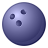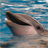# Calculating Field Values from multiple attribute tables

412
3
03-25-2013 08:37 AMNew Contributor III
I have an attribute table (let's call it table X) that I need to calculate field values for.
I have five additional attribute tables (lets call these tables A,B,C,D,E) that contain field 1. For each record in each table (A,B,C,D,E) I need to get an average for these five records and have that average recorded its corresponding field in table X.

For example looking at record ID1 in all tables, A=1 B=2 C=1 D=3 E=5
The value for record 1 in table X would = 2.4 ((1+2+1+3+5)/5))

The calculate field tool appears to only let you calculate a field value based on other fields in the same table. I need to calculate fields based on fields in other tables. Can I do this?
Tags (3)
3 RepliesNew Contributor III
Rachel,

Is there a common attribute between all of the tables?

If so you could join all of the tables together and use the calculate field tool and then remove the join.New Contributor III
Rachel,

Is there a common attribute between all of the tables?

If so you could join all of the tables together and use the calculate field tool and then remove the join.

There are common fields between the five tables that I need to average but not in the attribute table that I need the calculated value to go.

I'm thinking there might be a way to use update cursor to do this?byEsri Contributor
There are common fields between the five tables that I need to average but not in the attribute table that I need the calculated value to go.

I'm thinking there might be a way to use update cursor to do this?

You need some common attribute to join the values in A,B,C,D,E to a particular record in X. There has to be some way to link them together.  Joel's recommendation (Joins & Calc) is good. Update cursor is basically the same thing as a CalcField.